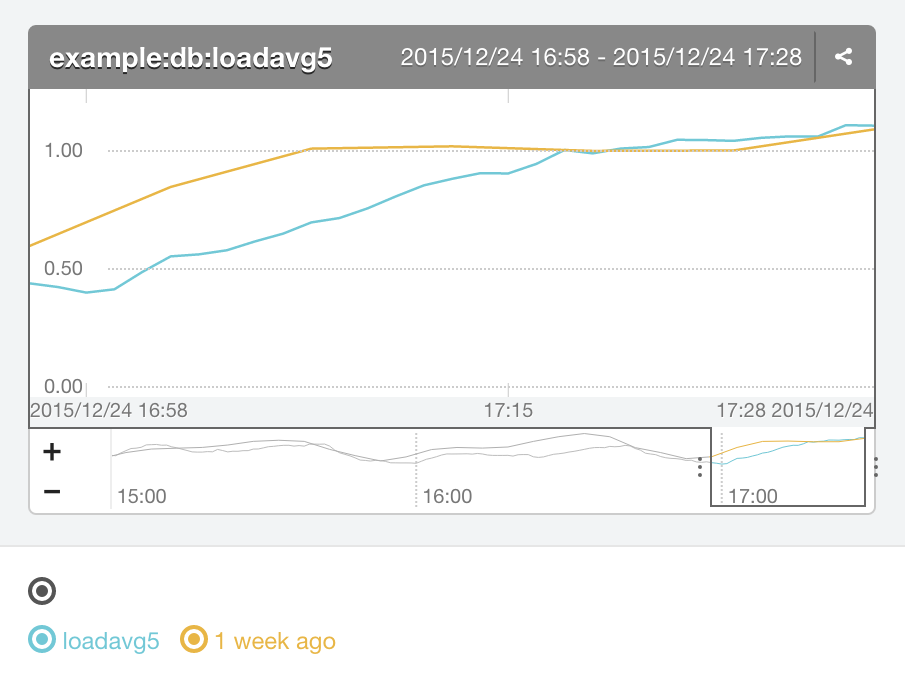# Displaying customized graphs

By writing a simple expression in query parameters for expanded graph permalink URLs, it's possible to display flexible, function-based graphs. (experimental feature)

Expanded graph permalinks can be used as demonstrated here `https://mackerel.io/orgs/<organization name>/advanced-graph?query=<metric>&unit=<unit>&title=<title>`. (title and unit parameters can be omitted)

## Example

### This is an overlay of an the average value of the `loadavg5` metric of the role `db` in the service `example` of the organization `example`, and the same metric from one week prior.

```query: group(alias(avg(role(example:db, loadavg5)), 'loadavg5'),
alias(timeShift(avg(role(example:db, loadavg5)), 1w), '1 week ago'))## Functions that can be used with expanded graph permalinks

Function Explanation Example
`host(hostId, metricName)` Returns host metrics. `host(22CXRB3pZmu, memory.*)`
`service(serviceName, metricName)` Returns service metrics. `service(Blog, access_count)`
`role(roleFullname, metricName)` Returns host metrics that currently belong to a role. `role(Blog:db, memory.*)`
`roleSlots(roleFullname, metricName)` Returns a role's metrics. Metrics sent from hosts that used to belong to a role in the past can also be gotten. Every custom metric and some system metrics*1 can be used. `roleSlots(Blog:db, loadavg5)`
`avg(metrics)` Returns an average of metrics of an argument for each point in time. `avg(group(host(22CXRB3pZmu, loadavg5), host(22CXRB3pZmu, loadavg5)))`
`max(metrics)` Returns the largest metric value of an argument for each point in time. `max(host(22CXRB3pZmu, custom.foo.jobs.*))`
`min(metrics)` Returns the smallest metric value of an argument for each point in time. `min(host(22CXRB3pZmu, custom.foo.jobs.*))`
`sum(metrics)` Returns the sum total of metrics of an argument for each point in time. `sum(host(22CXRB3pZmu, custom.foo.jobs.*))`
`product(metrics)` Returns the multiplied total of metrics of an argument for each point in time. `product(group(service(Blog, foo.bar), service(Blog, foo.baz)))`
`diff(metrics, metrics)` Returns the first metric minus the second metric of an argument for each point in time. `diff(service(Blog, foo.bar), service(Blog, foo.baz))`
`divide(metrics, metrics)` Returns the first metric divided by the second metric of an argument for each point in time. `divide(service(Blog, foo.bar), service(Blog, foo.baz))`
`scale(metrics, factor)` Returns metrics doubled a fixed number of times. `scale(service(Blog, foo.bar), 10.0)`
`offset(metrics, factor)` Returns metrics added a fixed number. `offset(service(Blog, foo.bar), 10.0)`
`percentile(metrics, percentage)` Returns the `percentage` percentile value of the metrics given as the argument for each point in time. `percentile(role(Blog:db, loadavg5), 80)`
`timeShift(metrics, duration)` Returns metrics shifted by a designated amount of time. `timeShift(service(Blog, foo.bar), 1d)`
`movingAverage(metrics, duration)` The average movement. `movingAverage(service(Blog, foo.bar), 1d)`
`linearRegression(metrics, duration)` Returns linear regression metrics based on the metric value from the current time until before `duration` `linearRegression(host(22CXRB3pZmu, loadavg5), 7d)`
`timeLeftForecast(metrics, duration, threshold)` Returns the number of seconds remaining until the linear regression value reaches the `threshold`, based on the metric value from the current time until before `duration` `scale(timeLeftForecast(host(22CXRB3pZmu, cpu.system.percentage), 7d, 10), 1/86400)`
`group(metrics, metrics, ...)` Compiles argument metrics into one line. `group(service(Blog, foo.bar), service(Blog, foo.baz))`
`stack(metrics)` Displays graph in stack mode. `stack(service(Blog, foo.bar))`
`alias(metrics, displayName)` Customizes a metric's display name. `alias(service(Blog, foo.bar), 'Blog foo bar')`

*1 `loadavg5`, `processor_queue_length`, `cpu.user.percentage`, `cpu.iowait.percentage`, `cpu.system.percentage`, `interface.rxBytes.delta`, `interface.txBytes.delta`, `disk.reads.delta`, `disk.writes.delta`, `memory.used`, `memory.cached`, `container.cpu.usage`, `container.memory.usage`

All functions will return metrics. The parameters of the functions' arguments are as follows.

Argument Type Explanation Example
hostId string host ID `22CXRB3pZmu`, `'22CXRB3pZmu'`, `"22CXRB3pZmu"`
metricName string metric name `loadavg5`, `'loadavg5'`
serviceName string service name `Blog`, `'Blog'`
roleFullname string service name and role connected with a `:` `Blog:db`, `'Blog:db'`
metrics metrics metrics line `alias(host(22CXRB3pZmu, loadavg5), 'L5')`
displayName string display name `'blog max loadavg5'`
duration string Duration is specified as an integer followed by the unit of time. Time units that can be used are m(minutes), h(hours), d(days), w(weeks), mo(months), and y(years). `5m`, `1d`
factor float factor `1.5`, `200`, `1/86400`
threshold float threshold `1.5`, `200`, `1/86400`
percentage float percentage (0 < `percentage` < 100) `80`, `99.9`